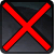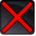# Nasty BiteConditions

## Used by

Used by
• Class 16141029303055434053

## Related effects, buffs and debuffs

• [0s] [not displayed]Effect #1

 Slot: Debuff Duration: 0s Tick rate: does not tick # occurrences: 0
• On Apply

Only when the following conditions are met:

• If difficulty is not set to Nightmare Mode

Perform the following actions:

• Play appearance epp . qtr . 1x4 . raid . asation . trash . station . larva . larva_chomp
• Spell Damage
- Unknown (609) = (bool) false
- Damage Type = (int) 2
- Slot = (int) 1
- Spell Type = (int) 3
- Level Cap = (int) 0
- Amount Max = (float) 0
- Amount Min = (float) 0
- Amount Percent = (float) 0
- Threat Percent = (float) 0
- Standard Health Percent Max = (float) 0.68
- Standard Health Percent Min = (float) 0.68
- Amount Modifier Percent = (float) 0
- Coefficient = (float) 1
- Health Steal Percentage = (float) 0
• On Apply

Only when the following conditions are met:

• If difficulty is set to Nightmare Mode

Perform the following actions:

• Play appearance epp . qtr . 1x4 . raid . asation . trash . station . larva . larva_chomp
• Spell Damage
- Unknown (609) = (bool) false
- Damage Type = (int) 2
- Slot = (int) 1
- Spell Type = (int) 3
- Level Cap = (int) 0
- Amount Max = (float) 0
- Amount Min = (float) 0
- Amount Percent = (float) 0
- Threat Percent = (float) 0
- Standard Health Percent Max = (float) 0.2266
- Standard Health Percent Min = (float) 0.2266
- Amount Modifier Percent = (float) 0
- Coefficient = (float) 1
- Health Steal Percentage = (float) 0
• On Apply

Only when the following conditions are met:

• If difficulty is set to Nightmare Mode

Perform the following actions:

• Spell Damage
- Unknown (609) = (bool) false
- Damage Type = (int) 2
- Slot = (int) 1
- Spell Type = (int) 3
- Level Cap = (int) 0
- Amount Max = (float) 0
- Amount Min = (float) 0
- Amount Percent = (float) 0
- Threat Percent = (float) 0
- Standard Health Percent Max = (float) 0.2266
- Standard Health Percent Min = (float) 0.2266
- Amount Modifier Percent = (float) 0
- Coefficient = (float) 1
- Health Steal Percentage = (float) 0
• On Apply

Only when the following conditions are met:

• If difficulty is set to Nightmare Mode

Perform the following actions:

• Spell Damage
- Unknown (609) = (bool) false
- Damage Type = (int) 2
- Slot = (int) 1
- Spell Type = (int) 3
- Level Cap = (int) 0
- Amount Max = (float) 0
- Amount Min = (float) 0
- Amount Percent = (float) 0
- Threat Percent = (float) 0
- Standard Health Percent Max = (float) 0.2266
- Standard Health Percent Min = (float) 0.2266
- Amount Modifier Percent = (float) 0
- Coefficient = (float) 1
- Health Steal Percentage = (float) 0